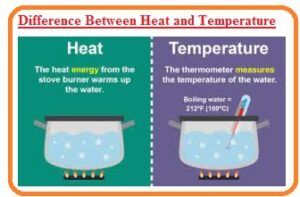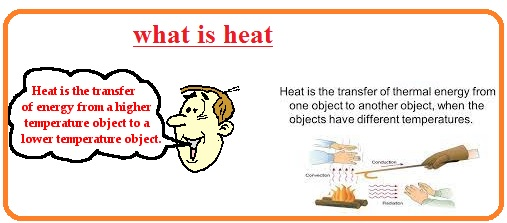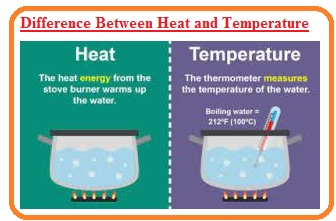Hi, readers welcome to the new post. in this post, we will have a detailed look at Difference Between Heat and Temperature. both of these are somewhat different but have some common relation to one another. Heat is type of energy and temperature discusses the quantity of thermal energy. The common relation among them is that with the larger value of heat there will rise in temperature.

With the decrement in heat temperature decreases Here we discuss the parameters of these two with detail. So let get started Difference Between Heat and Temperature.

## Difference Between Heat and Temperature

Introduction to Heat

• Heat define as a form of energy that is the sum of energy of all particles existing in the body.
• It is transferred from one part of the body to another due to a change in temperature.
• Heat transfer from hot to cool object. The units used for this are jouled calories.
• There are 3 common ways through which heat transferred first one is radiation second one is conduction and third one is convection.
• Heat transformation due to physical connection called conduction heat transfer due to movement of particles of objects from one location to another called convection and through radiation heat transfer in a vacuum.Introduction to Temperature

• It tells the degree of hotness and coldness of body and also define the sum of the kinetic energy of all particles of the body/
• As it is the average calculation so temperature value does not depend on the physical and quality of particles of the body.
• It used to find the speed of parties like atoms and molecules of body.
• The unit used to measure its value are Fahrenheit, Celsius, and kelvin.
• The thermometer is a device used to finds its value.## Comparison Between Heat and TemperatureWhat is Heat

• It is the form of energy and type of energy.
• It denoted as Q
• The ability to perform work also called heat.
• The units used for its measurements are joule and calorie.
• calorimeter used for its measurements.
• it is the net sum of kinetic and potential energy that occurs in objects.

What is Temperature

• Temperature explains the degree of hotness and coldness of the body.
• It is denoted with T.
• It helps to do the measurement of the quantity of heat.
• Units used for this are kelvin and centigrade.
• The thermometer is a device used for temperature measurements.
• It is the sum of the average K.E of molecules.

That is a detailed post about Difference Between Heat and Temperature if you has any further query ask in the comment. Thanks for reading have a nice day.